# Errors

The major systematic errors in this experiment are due to the heating effect, end corrections introduced due to shift of the zero of the scale at A and B, and stray resistances in P and Q, and errors due to non-uniformly of the meter bridge wire.

## Error Analysis

End corrections can be estimated by including known resistances P1and Q1 in the two ends and finding the null point: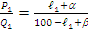(where a and b are the end corrections).

When the resistance Q1 is placed in the left gap and P1 in the right gap,which give two linear equation for finding a and b.

In order that aand b be measured accurately, P1 and Q1 should be as different from each other as possible.

For the actual balance point,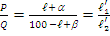Errors due to non-uniformity of the meter bridge wire can be minimized by interchanging the resistances in the gaps P and Q.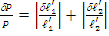Where d and d are the order of the least count of the scale.

The error is, therefore, minimum if=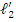, i.e. when the balance point is in the middle of the bridge.

The error in r is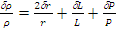To read more, Buy study materials of Current Electricity comprising study notes, revision notes, video lectures, previous year solved questions etc. Also browse for more study materials on Physics here.### Course Features

• Video Lectures
• Revision Notes
• Previous Year Papers
• Mind Map
• Study Planner
• NCERT Solutions
• Discussion Forum
• Test paper with Video Solution